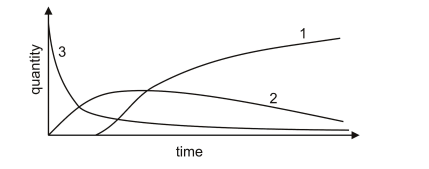One radioactive substance, P, is gradually changed by radioactive decay into another, Q. Q itself decays into a third substance, R, which does not decay. The graphs below show how the quantities of P, Q and R varied with time during an experiment.What do the graphs 1, 2 and 3 represent?

A. 1:R 2:Q 3:P
B. 1:Q 2:P 3:R
C. 1:Q 2:R 3:P
D. 1:P 2:Q 3:R
E. 1:R 2:P 3:Q

We notice that curve 3 is a decay curve, and so, we must ask ourselves which of the 3 substances has a normal radioactive decay. The answer would be P, as mentioned by the question itself.

We now know 3:P, which helps us narrow our answer down to either A or C.

Next, we can observe in curve 2 that there is a temporary increase, followed by a decrease.

Since the question clearly states that Q decays into R, which does not decay- we can affirm that R will only be constantly produced. Hence, if a substance is R, it should only increase in quantity.

Quickly we can come to 2 conclusions:

Curve 1 only increases in quantity, it surely is R.
Curve 2 will then be Q.

1:R, 2:Q, 3:P, making our answer A.